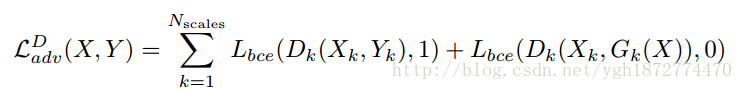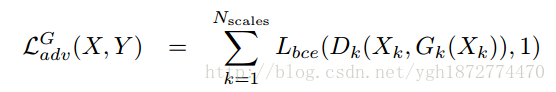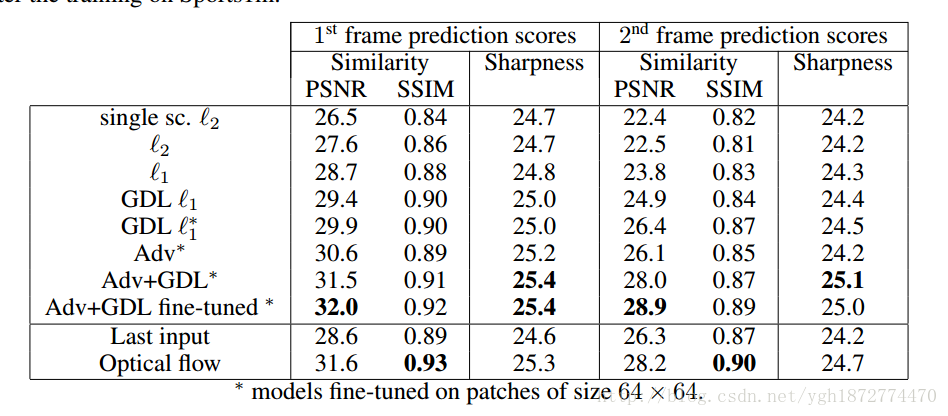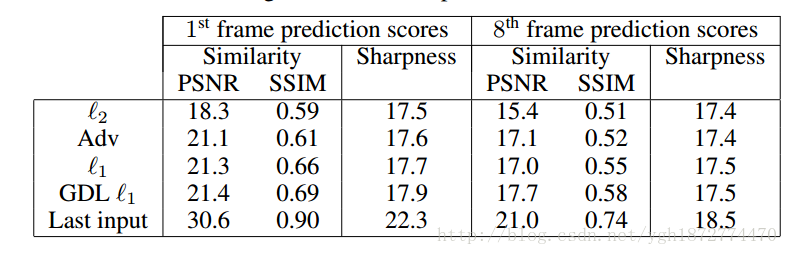# Video prediction beyond mean square error

Be prepared to blog about a series of papers for your own convenience.
This is the first one
DEEP MULTI-SCALE VIDEO PREDICTION BEYOND MEAN SQUARE ERROR
In this paper, I mainly focus on the prediction generated by gan network

First of all, our input includes real pre-frame X, real pre-predicted frame Y, generator G, discriminator D
. When training the discriminator, we package the real X and Y as (X,Y)
. When training the generator, we package X and gaussian distribution Y ‘to get (X,G(X)) and use it as the training of
discriminator in the input
input discriminator
For real images, we define D(X,Y)=1 and. For real images, we define D(X,G(X))=0Lbce stands for cross entropy
Generator training
for generating images, let D(X,G(X))=1Then the stochastic gradient descent method was used for training
Training network
generator, mapped to (m+n)*h*w-> (m+n)*h*w
discriminator, mapping to (m+n)*h*w-> [0, 1]
Experimental results:
data set: UCF101
1 frame and 2 frame prediction1 frame and 8 frame prediction